﻿ STUDY OF GREAT SPEEDS

### Home | Up one level | Previous | Next

WALTER RITZ

#### Annales 254 (Oeuvres 408)

The case where the speed of electrons is comparable to the speed of light is presented only

Annales 255

for β rays of radium. We have studied the deviability of these rays under the influence of an electrostatic field and under that of a magnetic field produced by electromagnets, that is neutral closed currents, whose electrons have speeds v' very small in relation to c. The accelerations always stay small in these experiments.
We can consequently develop again, in expression (VI) of the elementary force, the quantities r, u2,ur following the formulas of no 3, these expansions supposing small not the speeds but the accelerations of diverse orders. We will have, v being the speed comparable to c, whereas v'/c is very small,Let v/c=β be a new vector of length comparable to (Oeuvres 409) unity; we will have

(35)(36)(37)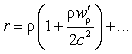In expression (VI) of Fx one can develop φ, ψ following Taylor’s formula in the setting of values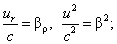since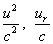differ from

Annales 256

these values only by very small quantities ε, η of the order of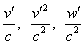, . . . ,we will have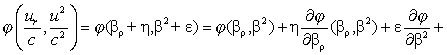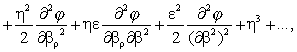(38)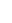(39)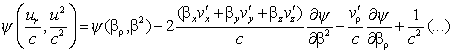The expression (VI) of Fx will thus become

(40)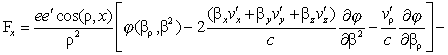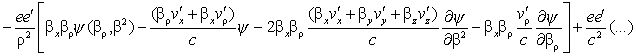(Oeuvres 410) The terms in 1/c2 will be negligible compared to those of the first order; for the electrostatic action (v'=0) it will simply become

(41)To obtain the action of an element ds' of a neutral

Annales 257

closed current whose electrostatic charge can be neglected, that is for which the positive and negative charges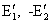are clearly equal by unity of volume and of opposite signs, we have only to add the sum of the actions of the positive and negative ions of ds' on the electron e; let v1' , v2'  be the speeds of the positive and negative ions, the conductor or magnet being at rest, we will have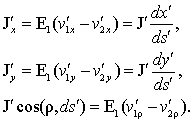In the sum of the actions of both kinds of ions on e, the terms independent of v' being taken with opposite signs are canceled in (40), and there remains

(42)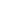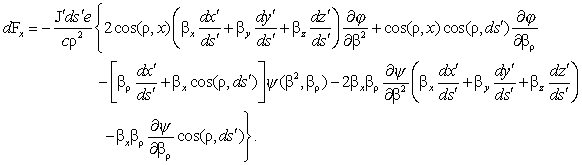The action of the current is thus proportional to its intensity. Furthermore, two elements of parallel current of opposite direction and of the same intensity have no action. If therefore we consider a uniformly magnetized magnetic sheet as being a system of very small closed currents of the same intensity, only the parts of these currents situated on the edge of the sheet will have a noticeable action; (Oeuvres 411) the effect of the currents situated at the interior will tend towards zero with the dimensions and the distances of the currents. Such a magnetic sheet will therefore be equivalent

Annales 258

to a closed current circulating on its contour: this is exactly the reasoning that one makes in electrodynamics, and it would be easy to give it a more rigorous form.

Similarly, F being a linear function of the cosine directionsof the current element, the principle of the sign currents is satisfied for J.

But there ends the analogy with classical electrodynamics. Thus for weak speeds (that is to say small β) we saw earlier that the action Fx, Fy, Fz of a closed current on a moving electron is perpendicular to the velocity of the latter. It is not so, in general, for β compared to unity. Indeed, the component R of F parallel to β will be

(43)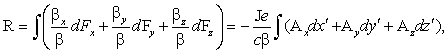where we put, to abbreviate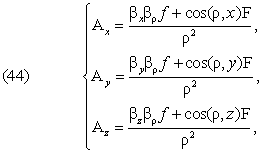The quantity R will be nil for all closed circuits only ifis a total differential, that is we have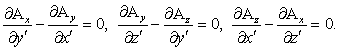Annales 259

(Oeuvres 412) But β2 is independent of x', y', z' and we have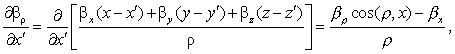.

The condition necessary and sufficient in order that the force be perpendicular to the speed will thus be that the expressions f, F, formed by means of φ, ψ, satisfy the differential equation

(46)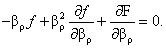For very small β, f is a constant f0 and F is equal to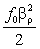; the relation is satisfied.

When φ and ψ do not depend on βρ, the relation take the simple form f=0.

Likewise, the action of a closed solenoid, or of a closed electromagnet, is not nil in general, unless a certain differential equation of the third order between φ and ψ is satisfied.

Finally, more generally, the knowledge of the magnetic field in a point is not sufficient to determine the force that an electron in very fast motion will experience at that point, unless the stated relations are satisfied. This last force is determined only when we give the disposition of currents (assuming the magnets being replaced by the equivalent currents) and by this the values of βρ for the diverse elements of current.

The experiment should thus first of all decide if the

Annales 260

several laws of electromagnetism just in question apply to the β rays of radium. Leaving the notion of field, these questions are not even asked and it is no doubt for this reason that, to my knowledge, no precise quantitative experiment has yet answered them. Kaufmann’s fine experiments, undertaken towards a different goal, do not allow answering them, as we are going to see.

On the whole, the notion of field will apply to the action that (Oeuvres 413) β rays undergo only under certain conditions; we have moreover shown that this notion introduces, in general (that is the forces and the field depending on the speeds), absolute motion. The ordinary effects of the magnetic field escape this rule only to a certain approximation (neglecting the motion of the Earth, etc…)